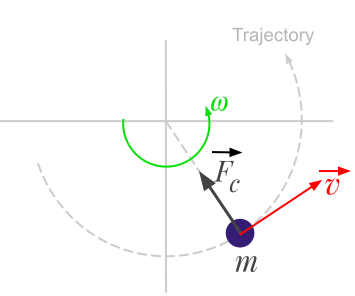👋 Hello! I'm Alphonsio the robot. Ask me a question, I'll try to answer.

# centripetal force

Centripetal force is a force that acts on all bodies moving along a circular path. This force is directed towards the center around which the body is moving. This force is associated with the centripetal acceleration (acceleration linked to the change of orientation of the speed vector): ﻿﻿ .Here are some examples of centripetal forces:

• A rotating athletic hammer: the cable exerts a force on the hammer to keep it at a constant distance, it is a centripetal force.
• The movement of the planets: the gravitational attraction exerted by the earth on the moon is a centripetal force. Without this force, the moon would follow a straight path at constant speed.
• A car in a bend: the frictional force between the wheels and the ground is a centripetal force.

The centripetal force is calculated using the following formulas:

﻿﻿

Where:

• ﻿﻿ is the centripetal force expressed in Newton (﻿﻿)
• ﻿﻿ is the body mass expressed in kilograms (﻿﻿)
• ﻿﻿ is the radius of the circular path expressed in meters (﻿﻿)
• ﻿﻿ is the linear velocity of the body expressed in meters per second (﻿﻿)
• ﻿﻿ is the radius of the circular path expressed in meters (﻿﻿)
• ﻿﻿ is the angular velocity of the body expressed in radians per second (﻿﻿)
More